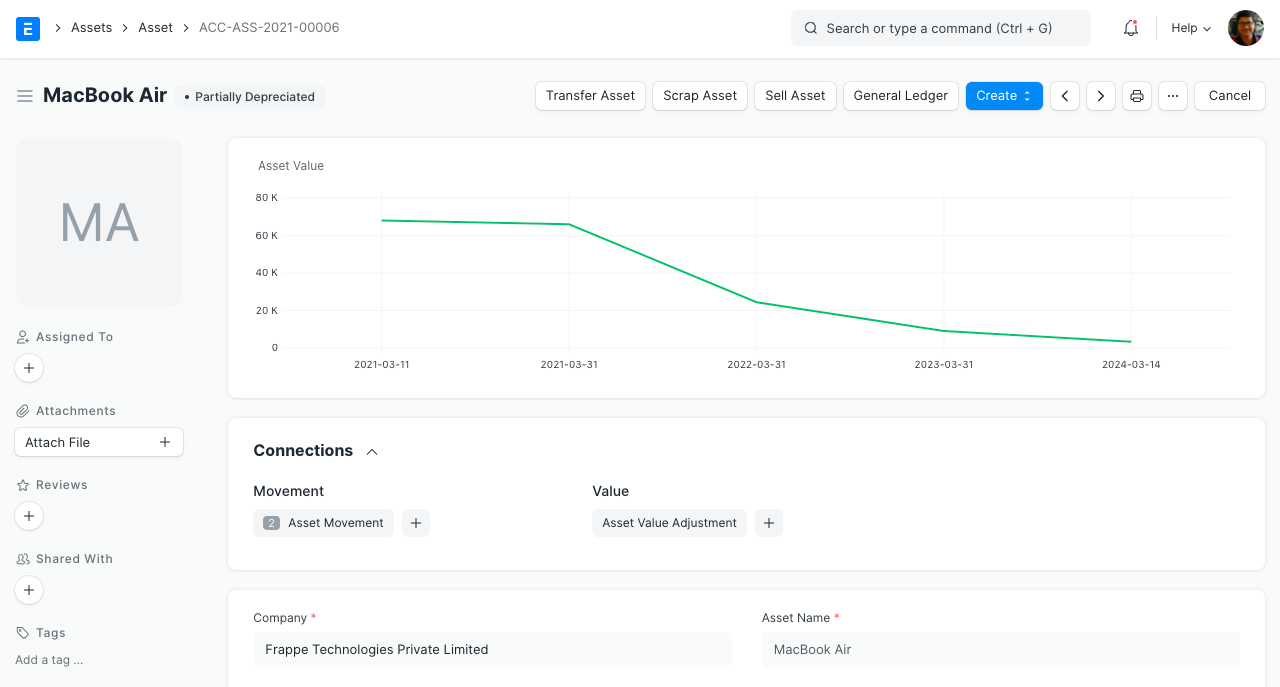# Asset Depreciation

The system automatically creates a depreciation schedule based on the `Depreciation Method`, `Total Number of Depreciations`, etc., and other related inputs like `Available to Use Date` in the Asset record. It is also possible to create multiple depreciation schedules for different Finance Books. You need to tick the `Calculate Depreciation` checkbox while creating an asset if you want the system to create the depreciation schedule.## Different depreciation methods in ERPNext:

• Straight line: In this method, the value of an asset is reduced uniformly over its useful life until it reaches its salvage value. For example: if the asset is worth 1000 and its salvage value is 500 after 5 years, the straight line method would depreciate the asset by 100 every month/year. This method is useful when there is no particular pattern to how the depreciation takes place over a period of time. You can use the 'Daily Depreciation' option if you want the depreciation amount to vary depending on the number of days in the month.

• Double Declining Balance: This is also known as 200% declining balance. This method is useful when the asset depreciates fast in the beginning and slows down later. For example: if the asset is worth 1,00,000 and its salvage value is 11,000 after 8 years, the depreciation schedule would be:

Current Value

Depreciation

Booked Value

1,00,000.00

25,000.00

75,000.00

75,000.00

18,750.00

56,250.00

56,250.00

14,063.00

42,187.50

42,187.50

10,547.00

31,640.62

31,640.62

7,910.00

23,730.46

23,730.46

5,933.00

17,797.84

17,797.84

4,449.00

13,348.38

13,348.38

2,348.00

11,000.00

• Written Down Value: In this method, you can set a particular `rate of depreciation` or let the system calculate the `rate of depreciation` based on the asset’s purchase amount, salvage value and useful life. The `rate of depreciation` is applied on the current written down value of the asset to calculate the depreciation amount for each year. This method is useful for assets which have higher depreciation in the initial years. For example: if the asset’s purchase amount is 1,000 and `rate of depreciation` is 10% over 5 years, the depreciation schedule would be:

Current Value

Depreciation

Booked Value

1,000.00

100.00

900.00

900.00

90.00

810.00

810.00

81.00

729.00

729.00

72.90

656.10

656.10

65.61

590.49

• Manual: In this method, first a system-generated depreciation schedule would be created for your convenience based on the depreciation details set, and you can then edit the schedule dates and depreciation amounts manually for any period according to your needs.

## Automatic depreciation entries

You can enable booking of depreciation entry automatically from Accounts Settings. This will create depreciation entries automatically on the scheduled dates. Otherwise, you have to create the Journal Entry manually by clicking "Make Depreciation Entry" button in the corresponding Depreciation Schedule row.## Accounting entries on depreciation

In the depreciation entry:

• "Accumulated Depreciation Account" is credited and

• "Depreciation Expense Account" is debited.

The related accounts can be set in the Asset Category or Company.

## Asset value chart

For better understanding, net value of the asset on different depreciation dates are shown in a line graph.